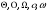# Name: cisc 3220 – sample exam 1

 Date 28.05.2018 Size 21.14 Kb.
Name: ____________________________________

CISC 3220 – SAMPLE EXAM 1

For maximum partial credit, show all your work clearly. Be sure your final answers are clearly marked. You may work on the backs of the pages, but indicate that you are doing so.

## Question 1 Time Complexity and Asymptotic Order (25 pts)

(10 pts) Part 1: Express the total time T(n) required by the following function. (Note: This would be the same as giving a formula for the value r returned by this function.)
Then, state the Theta-class of T(n).
int func(int n)

{

int r=0;

int i,j,k;

for (i=1; i<=n; i++)

for (j=i+1; j<=n; j++)

for (k=j+1; k<=n; k++)

r++;
return r;

}

##### (15 pts) Part II State the relationship between the functions f and g in each of the following in terms of. Be sure to point out where more than one relationship applies. Justify each answer with a few words.

(a) f(n)=log2 n g(n) = log10 n

(b) f(n) = n1/2 g(n) = n1/3

(c) f(n) = log2 n g(n) = n1/4

(d) f(n) = n7 – 7n5 g(n) = n5 + n

Question #2: Recurrence Equations (25 points)
Given the following recurrence equation:
T(1) = 1

T(n) = 8T(n/2) + n3
(a) Draw the top three levels of the recursion tree for T(n). (Use … where it is clear)

(b) How many levels are there in the recursion tree?

(c) How much work is done at each level?

(d) Use the Master Theorem to solve for the Theta-class of T(n). Then, give one sentence to explain how you would be able to arrive at the same solution using the recursion tree.

Question #3: Searching (10 points)
Suppose that you are given an array A of size n, in which n is very large. You are told that only the first m entries of the array contain positive integers and the rest of the array is filled with all zeros. The assumption is that m is much smaller than n, however, you do not know that value of m.
Give an efficient algorithm that will determine the value of m. Analyze the worst case time complexity of your algorithm. (Be careful to use m and n appropriately in the analysis.)

Question #4: Lower Bounds (15 points)
Circle TRUE or FALSE for each of the following.
(a) It is impossible to give an algorithm that has a higher time complexity than the lower

bound for the given problem.

TRUE FALSE
(b) An algorithm is optimal if the lower bound for the problem equals the worst case time complexity for the algorithm.
TRUE FALSE
(c) A decision tree can be constructed for any algorithm that performs a comparison-based search (even one that has not yet been discovered.)
TRUE FALSE
(d) The average case time complexity is always better than the worst case time complexity.
TRUE FALSE

(e) The binary search is an optimal algorithm for the problem of searching a sorted array.

TRUE FALSE

Question #5: Sorting (20 points)
Suppose that you would like to run code for MergeSort on a given array A of n elements.

However, you are told that on your machine you are limited to declaring extra space for at most n/2+c elements. (c is a constant, and provides enough space for individual variable declaration.)

(a) Which part of the MergeSort algorithm will have difficulty with the space constraint, and why?

(b) In which level(s) of recursion will the MergeSort algorithm have to deal with the issue of the space constraint?

(c) How can you modify the algorithm to work around the space constraint? Is the asymptotic worst-case time complexity of MergeSort affected by this change?

Work Area